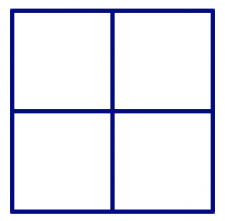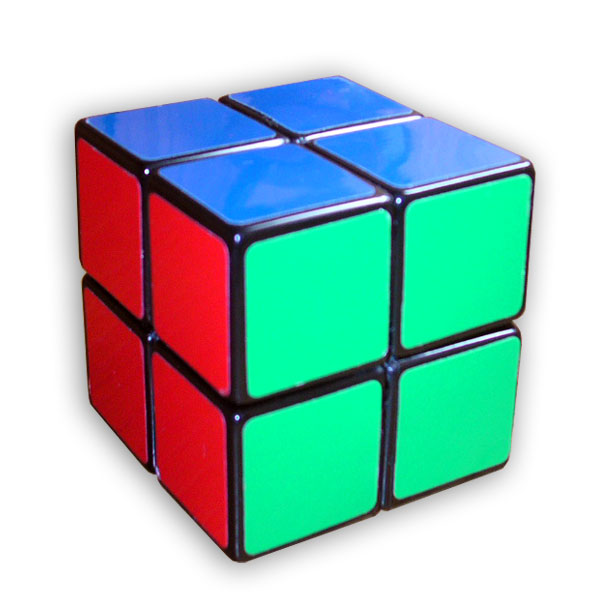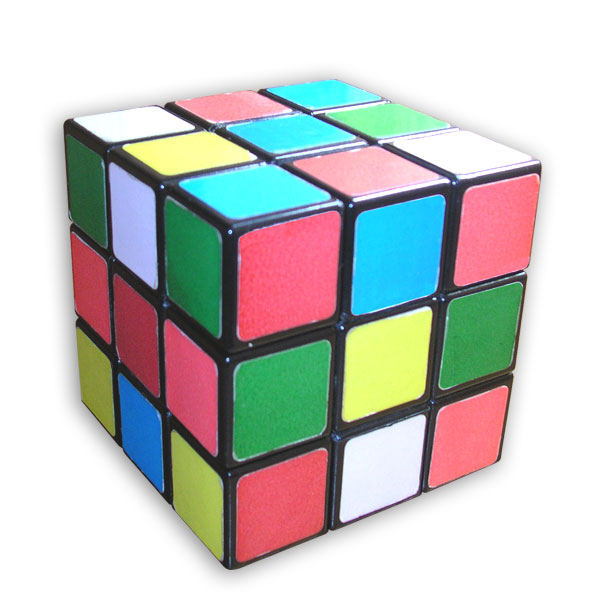$$\newcommand{\id}{\mathrm{id}}$$ $$\newcommand{\Span}{\mathrm{span}}$$ $$\newcommand{\kernel}{\mathrm{null}\,}$$ $$\newcommand{\range}{\mathrm{range}\,}$$ $$\newcommand{\RealPart}{\mathrm{Re}}$$ $$\newcommand{\ImaginaryPart}{\mathrm{Im}}$$ $$\newcommand{\Argument}{\mathrm{Arg}}$$ $$\newcommand{\norm}{\| #1 \|}$$ $$\newcommand{\inner}{\langle #1, #2 \rangle}$$ $$\newcommand{\Span}{\mathrm{span}}$$

# 7.6: Painted Cubes

•• Contributed by Michelle Manes
• Professor (Mathematics) at University of Hawaii
$$\newcommand{\vecs}{\overset { \rightharpoonup} {\mathbf{#1}} }$$ $$\newcommand{\vecd}{\overset{-\!-\!\rightharpoonup}{\vphantom{a}\smash {#1}}}$$$$\newcommand{\id}{\mathrm{id}}$$ $$\newcommand{\Span}{\mathrm{span}}$$ $$\newcommand{\kernel}{\mathrm{null}\,}$$ $$\newcommand{\range}{\mathrm{range}\,}$$ $$\newcommand{\RealPart}{\mathrm{Re}}$$ $$\newcommand{\ImaginaryPart}{\mathrm{Im}}$$ $$\newcommand{\Argument}{\mathrm{Arg}}$$ $$\newcommand{\norm}{\| #1 \|}$$ $$\newcommand{\inner}{\langle #1, #2 \rangle}$$ $$\newcommand{\Span}{\mathrm{span}}$$ $$\newcommand{\id}{\mathrm{id}}$$ $$\newcommand{\Span}{\mathrm{span}}$$ $$\newcommand{\kernel}{\mathrm{null}\,}$$ $$\newcommand{\range}{\mathrm{range}\,}$$ $$\newcommand{\RealPart}{\mathrm{Re}}$$ $$\newcommand{\ImaginaryPart}{\mathrm{Im}}$$ $$\newcommand{\Argument}{\mathrm{Arg}}$$ $$\newcommand{\norm}{\| #1 \|}$$ $$\newcommand{\inner}{\langle #1, #2 \rangle}$$ $$\newcommand{\Span}{\mathrm{span}}$$

You can build up squares from smaller squares:1 × 1 square 2 × 2 square 3 × 3 square

In a similar way, you can build up cubes from smaller cubes:1 × 1 × 1 cube 2 × 2 × 2 cube 3 × 3 × 3 cube

Think / Pair / Share

We call a 1 × 1 × 1 cube a unit cube.

• How many unit cubes are in a 2 × 2 × 2 cube?
• How many unit cubes are in a 3 × 3 × 3 cube?
• How many unit cubes are in a n × n × n cube?

Problem 10

Imagine you build a 3 × 3 × 3 cube from 27 small white unit cubes. Then you take your cube and dip it into a bucket of bright blue paint. After the cube dries, you take it apart, separating the small unit cubes.

1. After you take the cube apart, some of the unit cubes are still all white (no blue paint). How many? How do you know you are right?
2. After you take the cube apart, some of the unit cubes have blue paint on just one face. How many? How do you know you are right?
3. After you take the cube apart, some of the unit cubes have blue paint on two faces. How many? How do you know you are right?
4. After you take the cube apart, some of the unit cubes have blue paint on three faces. How many? How do you know you are right?
5. After you take the cube apart, do any of the unit cubes have blue paint on more than three faces? How many? How do you know you are right?

Problem 11

Generalize your work on Problem 10. What if you started with a 2 × 2 × 2 cube? Answer the same questions. What about a 4 × 4 × 4 cube? How about an n × n × n cube? Be sure to justify what you say.

1. Image by Robert Webb's Stella software: http://www.software3d.com/Stella.php, via Wikimedia Commons.
2. Image by Mike Gonzalez (TheCoffee) (Work by Mike Gonzalez (TheCoffee)) [CC BY-SA 3.0], via Wikimedia Commons.
3. Image by Mike Gonzalez (TheCoffee) (Work by Mike Gonzalez (TheCoffee)) [CC BY-SA 3.0], via Wikimedia Commons.